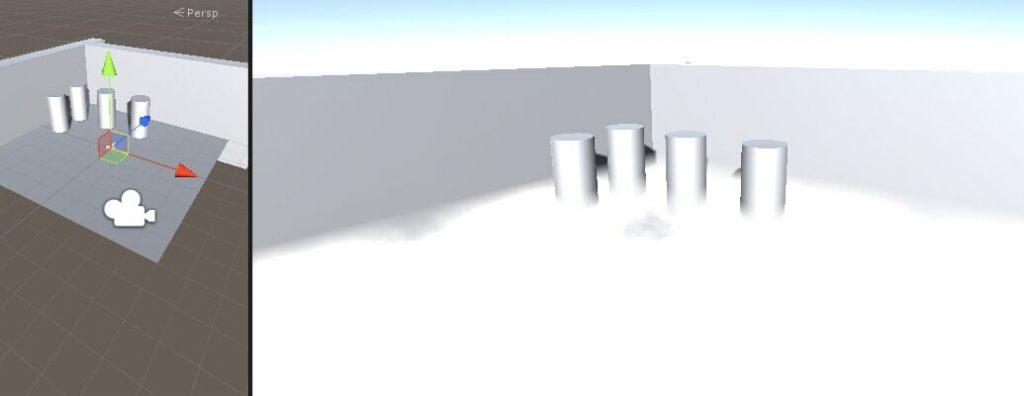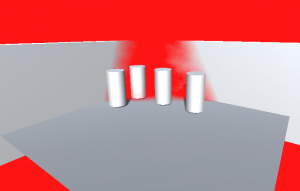# Unity3D 中的高度雾unity3d 一直都有一个距离雾，也可以说是深度雾，随着物体的远近慢慢渐变颜色，设置在 Window->Lightning->Fog(Unity 5.x)

Camera.main.depthTextureMode |= DepthTextureMode.Depth;

_FogStart 雾效在世界坐标系Y轴的开始值
_FogEnd 雾效在世界坐标系Y轴的结束值
_FogColor 雾效颜色

half diff = (_FogEnd – worldPos.y) / (_FogEnd – _FogStart);
fixed4 col = tex2D(_MainTex, i.uv);
fixed4 finalCol = lerp(col, _FogColor, diff);
return finalCol;```float2 center = float2(0.5, 0.5);
float2 pixelDir = normalize(i.uv - center);
```

```        Camera cam = Camera.main;
Transform CamTrans = cam.transform;
float near = cam.nearClipPlane;
float far = cam.farClipPlane;
float halfHeight = cam.nearClipPlane * Mathf.Tan(cam.fieldOfView * 0.5f * Mathf.Deg2Rad);
Vector3 toRight = CamTrans.right * halfHeight * cam.aspect;
Vector3 upVector = CamTrans.up * halfHeight;
Vector3 topVector = CamTrans.forward * near + upVector;
Vector3 bottomVector = CamTrans.forward * near - upVector;

Vector3 bottomLeft = bottomVector - toRight; //左下
Vector3 bottomRight = bottomVector + toRight; //右下
Vector3 topLeft = topVector - toRight; //左上
Vector3 topRight = topVector + toRight; //右上

```

```                               if (o.uv.y < 0.5 && o.uv.x < 0.5) {
}
else if (o.uv.y < 0.5 && o.uv.x > 0.5) { //右下
}
else if (o.uv.y > 0.5 && o.uv.x < 0.5) { //左上
```float3 worldPos = _WorldSpaceCameraPos + realDepth * i.cameraDir.xyz;